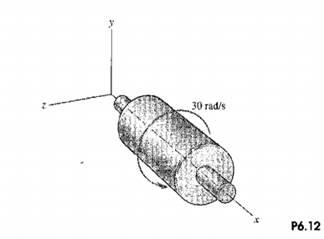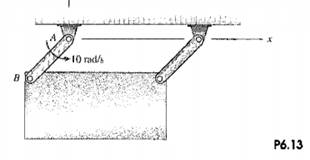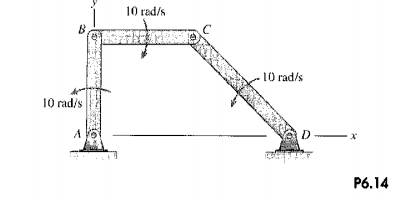### Create an Account

Already have account?

### Forgot Your Password ?

Home / Questions / 1 A turbine rotates at 30rads about a fixed axis coincident with the x axis What is its an...

# 1 A turbine rotates at 30rads about a fixed axis coincident with the x axis What is its angular velocity vector 2 The rectangular plate swings from arms of equal length Determine the angular

1. A turbine rotates at 30rad/s about a fixed axis coincident with the x axis. What is its angular velocity vector?2. The rectangular plate swings from arms of equal length, Determine the angular velocity&#39; vector of (a) the rectangular plate; (b) the bar AB, y3. What are the angular velocity vectors of each bar of the linkage?Jun 16 2020 View more View Less

#### Answer (Solved)Subscribe To Get Solution